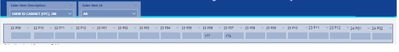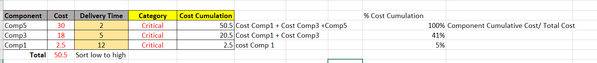cancel
Showing results for
Did you mean:

Fabric is Generally Available. Browse Fabric Presentations. Work towards your Fabric certification with the Cloud Skills Challenge.Helper I

## Dynamic value calculation based on input

Hi,

I have below scenario, I have been tried more than a month to get the solution but still not successful :in above table the total = 351 and I put in example 35004

User select Sale Item & enter Quantity in respective month input box (direct manual input in PBI report) .

The sale item has a set of component (~ 100 component).Yellow columns are dynamic value based on Input demand (sum of user input quantity).

How I am going to calculate total cost of critical component (this will change based on user enter value and sale item they selected)?

1 ACCEPTED SOLUTIONSuper User

``````Total Cost of Critical =
VAR T1 =
VALUES ( 'Table'[Component] ),
"@Cost", CALCULATE ( SELECTEDVALUE ( 'Table'[Cost] ) ),
"@Category", [Category]
)
VAR T2 =
FILTER ( T1, [@Category] = "Critical" )
RETURN
SUMX ( T2, [@Cost] )``````
9 REPLIES 9Helper I

Comp3 Cost 18

Comp5 Cost 30

Comp1 cost 2.5Helper I

```Total Cost of Critical =
VAR T1 =
VALUES ( 'Table'[Component] ),
"@Cost", CALCULATE ( SELECTEDVALUE ( 'Table'[Cost] ) ),
"@Category", [Category]
)
VAR T2 =
FILTER ( T1, [@Category] = "Critical" )
RETURN
SUMX ( T2, [@Cost] )```

Hi @tamerj1

Is it possible to calculate cost cumulation for Delivery Time lowest to higest?
I tried this formula but seem not work, it give the the Cost value of the component.
CALCULATE (SUMX(T2, [@Cost]). FILTER(ALL('Table'[Delivery Time]). 'Table'[Delibery Time]>=MIN('Table[Delivery Time])))

I am want result like below:

 Cost Delivery Time Cost Cummulation Comp3 18 5 50.5 Comp5 30 5 32.5 Comp1 2.5 12 2.5Super User

Sorry I did not understand the question. Would you please clarify further perhaps more details and some screenshots.Helper IAfter we get total Cost of Critical Category from previous formula, i want to calculate cost cumulation using delivery time low to high

What i did before( without considering category)

1.
Cost_Cumulation =
CALCULATE(SUM'Table1'[Cost], ALL('Table1'[Component]),
FILTER(ALL('Table'[Delivery Time),AND('Table'[Delivery Time >=MIN('Table'[Delivery Time]), NOT(ISBLANK('Table'[Delivery Time])))))
2.
% Cost Cumulation = [Cost Cumulation]/ CALCULATE([Cost Cumulation], 'Table1'[Delivery Time]=0)

Now the calculation need to consider Critical category only.Super User

``````Cumulative Cost of Critical =
VAR CurrentDeliveryTime =
SELECTEDVALUE ( 'Table'[Delivery Time] )
VAR T1 =
ALLSELECTED ( 'Table'[Component] ),
"@Cost", CALCULATE ( SELECTEDVALUE ( 'Table'[Cost] ) ),
"@Category", [Category],
"@DeliveryTime", CALCULATE ( SELECTEDVALUE ( 'Table'[Delivery Time] ) )
)
VAR T2 =
FILTER (
T1,
[@Category] = "Critical"
&& [@DeliveryTime] >= CurrentDeliveryTime
)
RETURN
SUMX ( T2, [@Cost] )``````Helper I

Hi @tamerj1

But Percentage & Category need to be calculated first because it based on quantity input total that user entered.Super User

What results didi you get using this proposed formula?Helper I

Hi @tamerj1

Ok, I know what you means.. I have already have category measure and just use that in formula.
I have tried your formula and it works... thank you soo much..  😄Super User

``````Total Cost of Critical =
VAR T1 =
VALUES ( 'Table'[Component] ),
"@Cost", CALCULATE ( SELECTEDVALUE ( 'Table'[Cost] ) ),
"@Category", [Category]
)
VAR T2 =
FILTER ( T1, [@Category] = "Critical" )
RETURN
SUMX ( T2, [@Cost] )``````Announcements#### Power BI Monthly Update - November 2023

Check out the November 2023 Power BI update to learn about new features.#### Fabric Community News unified experience

Read the latest Fabric Community announcements, including updates on Power BI, Synapse, Data Factory and Data Activator.#### The largest Power BI and Fabric virtual conference

130+ sessions, 130+ speakers, Product managers, MVPs, and experts. All about Power BI and Fabric. Attend online or watch the recordings.Top Solution Authors
Top Kudoed Authors
Users online (1,910)